Question

# Refer to Fig. P10-6. Determine the total elongation of strut AB due to a weight W=...

Refer to Fig. P10-6. Determine the total elongation of strut AB due to a weight W= 15 kN if the strut has a 10-mm diameter and is made of 300 mm steel with E 210 GPa.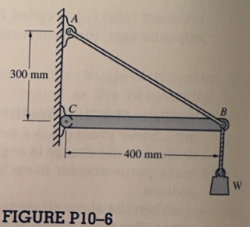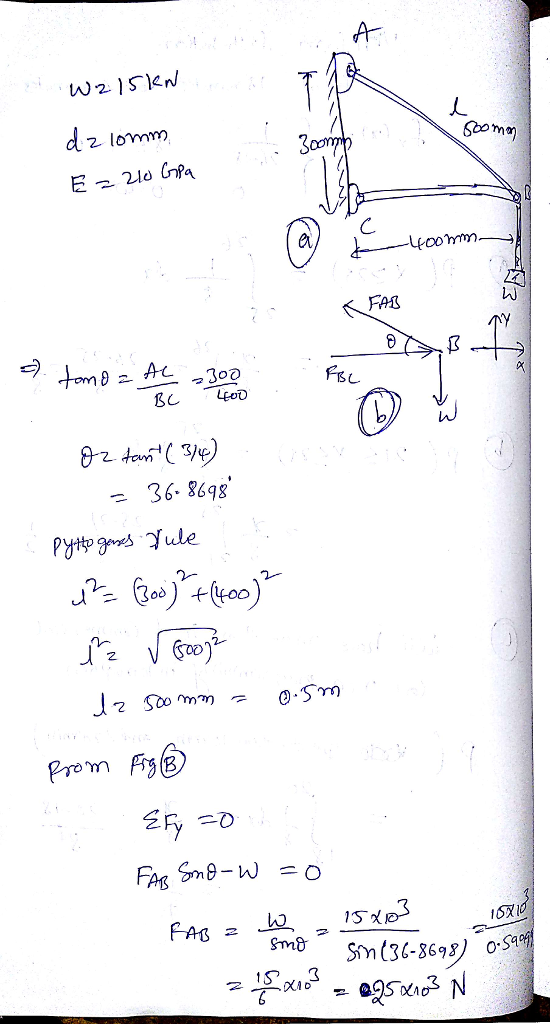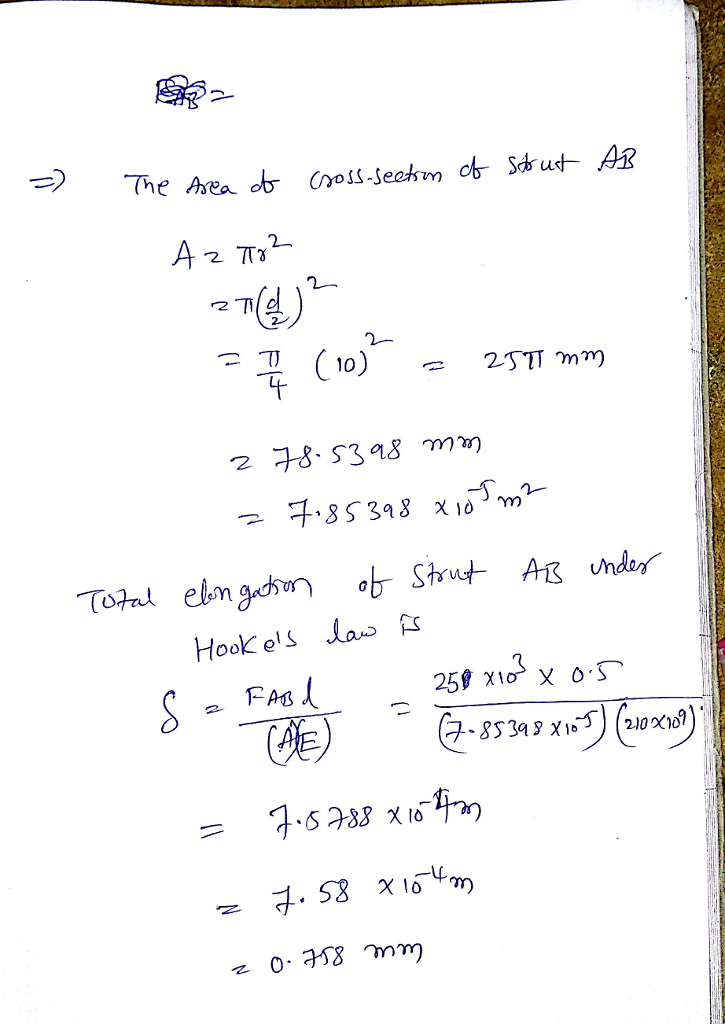#### Earn Coins

Coins can be redeemed for fabulous gifts.

Similar Homework Help Questions
• ### Modulus of Elasticity, Stress and Strain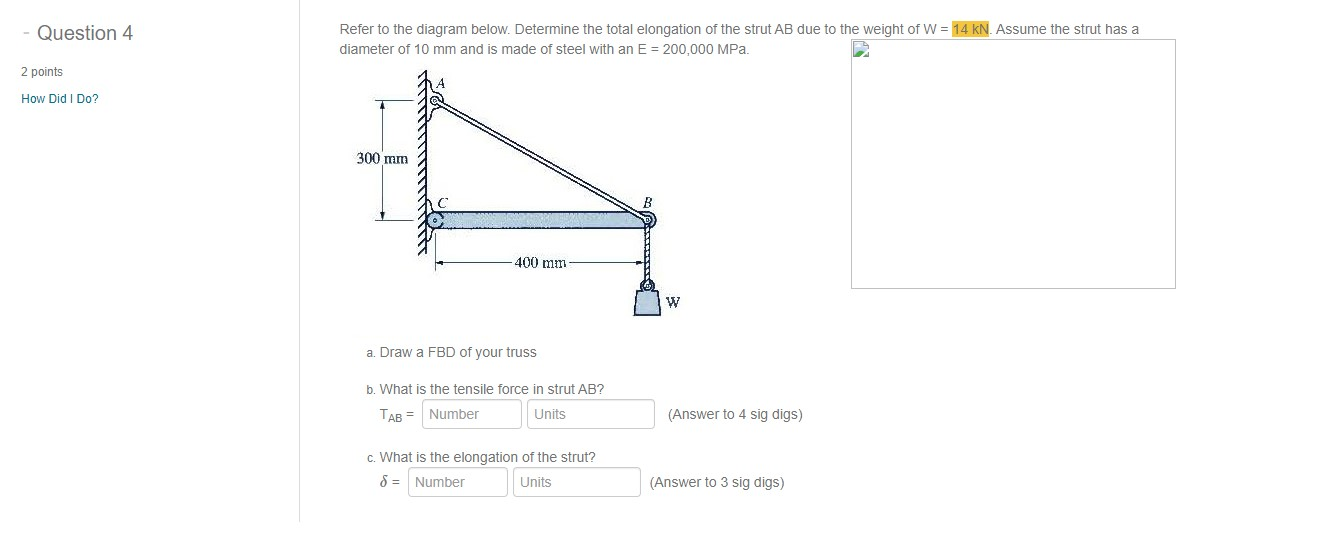Refer to the diagram below. Determine the total elongation of the strut AB due to the weight of W = 14 kN. Assume the strut has a diameter of 10 mm and is made of steel with an E = 200,000 MPa.

• ### stion 1 Determine the total vertical displacement of joint A (due to the load and tempreature...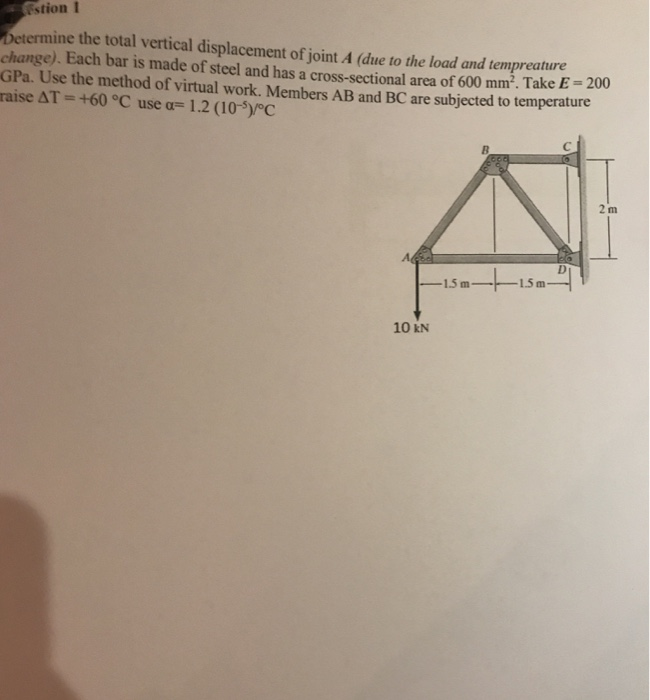stion 1 Determine the total vertical displacement of joint A (due to the load and tempreature change). Each bar is made of steel and has a cross-sectional area of 600 mm². Take E - 2001 GPa. Use the method of virtual work. Members AB and BC are subjected to temperature raise AT = +60 °C use a= 1.2 (10-5)/°C —15 m- 1.5m 10 KN

• ### 9.18 Two solid cylindrical rods are joined at B and loaded as shown AB is made...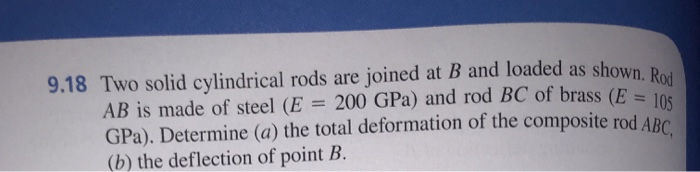9.18 Two solid cylindrical rods are joined at B and loaded as shown AB is made of steel (E = 200 GPa) and rod BC of brass (E = 1 GPa). Determine (a) the total deformation of the composite rod ARC (b) the deflection of point B. P = 30 KN + 30 mm 250 mm 40 KN 300 mm -50 mm Fig. P9.18

• ### A tie rod (1) and a pipe strut (2) are used to support a 50-kN load,...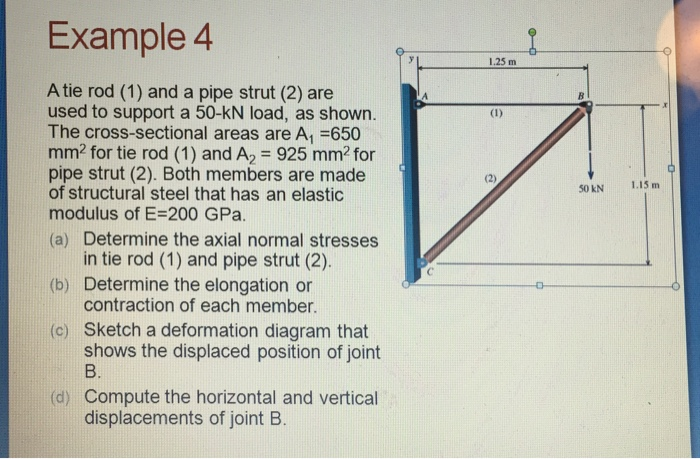A tie rod (1) and a pipe strut (2) are used to support a 50-kN load, as shown. The cross-sectional areas are A, =650 mm? for tie rod (1) and A_2 = 925 mm? for pipe strut (2). Both members are made of structural steel that has an elastic modulus of E = 200 GPa. (a) Determine the axial normal stresses in tie rod (1) and pipe strut (2). (b) Determine the elongation or contraction of each member. (c) Sketch...

• ### Q.1. (10 Marks) The rigid bar AB is supported by the pin-connected rod BC that has...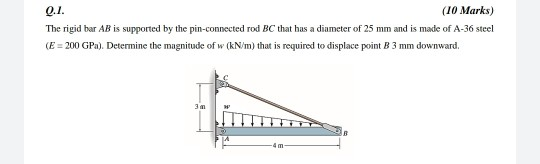Q.1. (10 Marks) The rigid bar AB is supported by the pin-connected rod BC that has a diameter of 25 mm and is made of A-36 steel (E = 200 GPa). Determine the magnitude of w (kN/m) that is required to displace point B 3 mm downward, 3

• ### A tie rod (1) and a pipe strut (2) are used to support a 50-kN load,...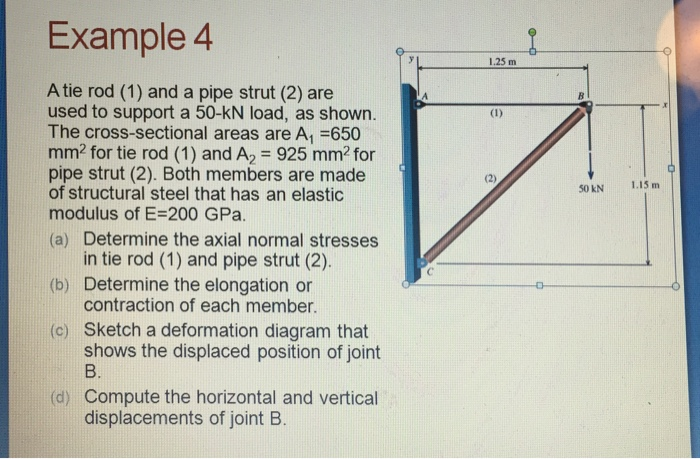A tie rod (1) and a pipe strut (2) are used to support a 50-kN load, as shown. The cross-sectional areas are A1 =650 mm2 for tie rod (1) and A2 = 925 mm2 for pipe strut (2). Both members are made of structural steel that has an elastic modulus of E=200 GPa. a) Determine the axial normal stresses in tie rod (1) and pipe strut (2). b) Determine the elongation or contraction of each member. c) Sketch a deformation...

• ### Q1. A circular rod of 12 mm diameter was tested for tension. The total elongation on...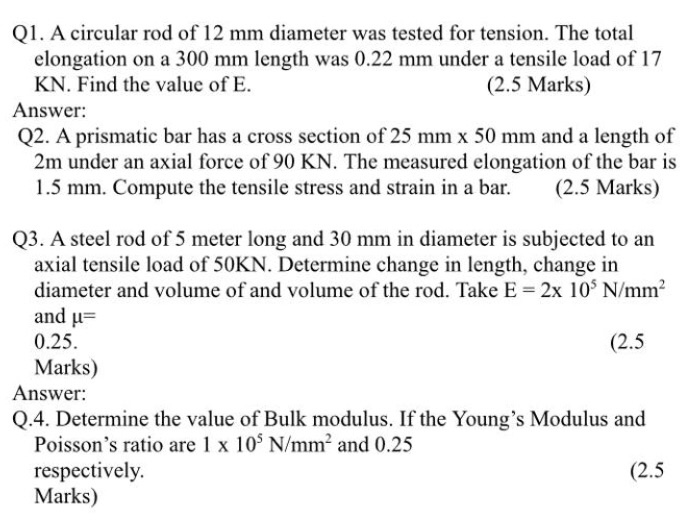Q1. A circular rod of 12 mm diameter was tested for tension. The total elongation on a 300 mm length was 0.22 mm under a tensile load of 17 KN. Find the value of E. (2.5 Marks) Answer: Q2. A prismatic bar has a cross section of 25 mm x 50 mm and a length of 2m under an axial force of 90 KN. The measured elongation of the bar is 1.5 mm. Compute the tensile stress and strain in...

• ### A rigid beam AB is supported by a hinge at A and by a steel wire...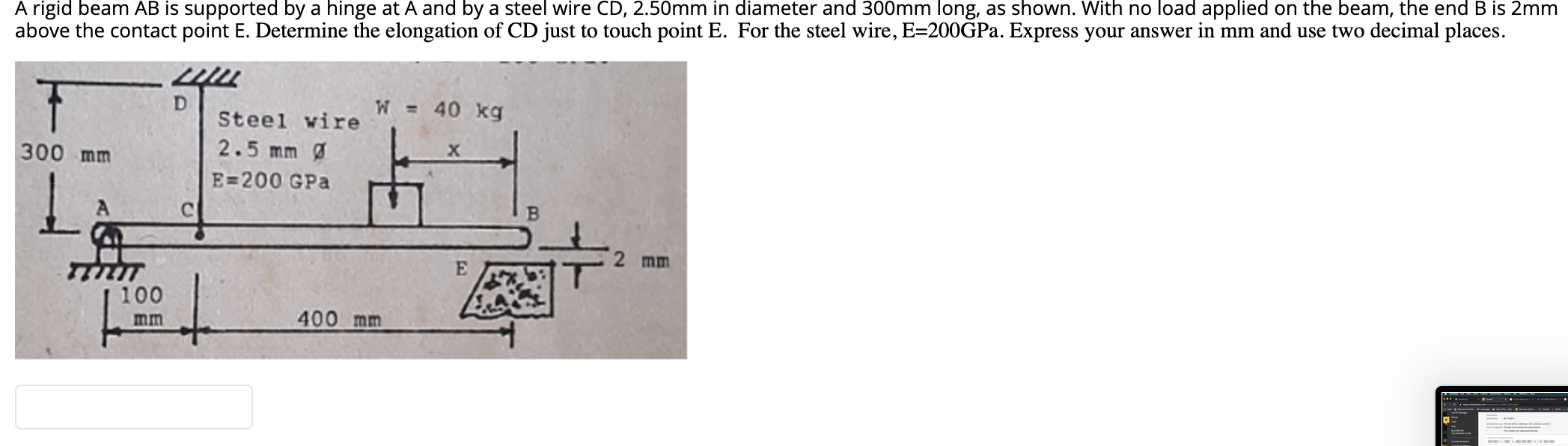A rigid beam AB is supported by a hinge at A and by a steel wire CD, 2.50mm in diameter and 300mm long, as shown. With no load applied on the beam, the end B is 2mm above the contact point E. Determine the elongation of CD just to touch point E. For the steel wire, E=200GPa. Express your answer in mm and use two decimal places. D W = 40 kg 44 Steel wire 2.5 mm E=200 GPa 300...

• ### Problem 6 А 300 mm di B 50 KN 250 mm d2 C P steel Section...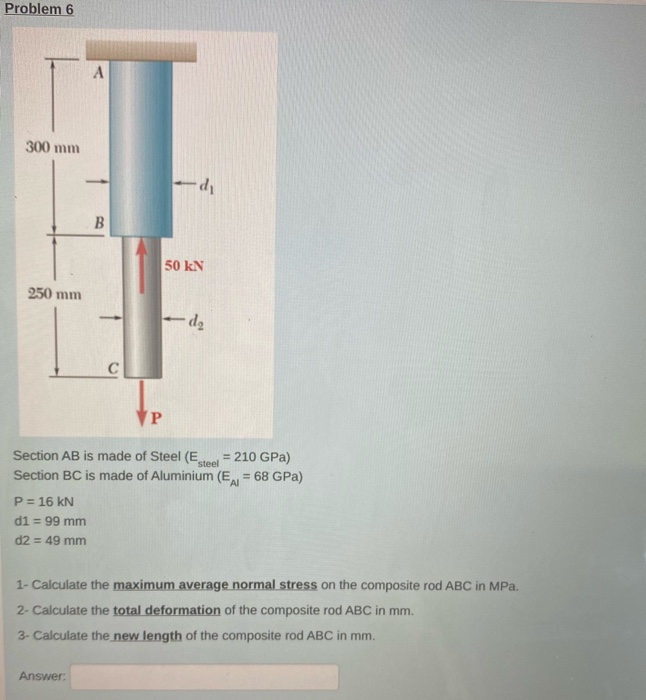Problem 6 А 300 mm di B 50 KN 250 mm d2 C P steel Section AB is made of Steel (E = 210 GPa) Section BC is made of Aluminium (EAL = 68 GPa) P = 16 KN d1 = 99 mm d2 = 49 mm 1- Calculate the maximum average normal stress on the composite rod ABC in MPa. 2- Calculate the total deformation of the composite rod ABC in mm. 3- Calculate the new length of the...

• ### Question 2 1.7-10 A metal bar AB of weight W is suspended by a system of...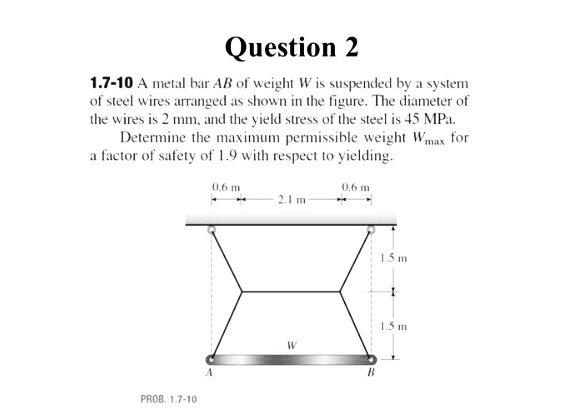Question 2 1.7-10 A metal bar AB of weight W is suspended by a system of steel wires arranged as shown in the figure. The diameter of the wires is 2 mm, and the yield stress of the steel is 45 MPa Determine the maxium permissible weight Wmax for a factor of safety of 1.9 with respect to yielding 0.6 m 0,6 m 2.1 m 15 m 1.5 m PROB. 1.7-10